# (1 point) Suppose that the matrix A has the following eigenvalues and eigenvectors: A1 = 4...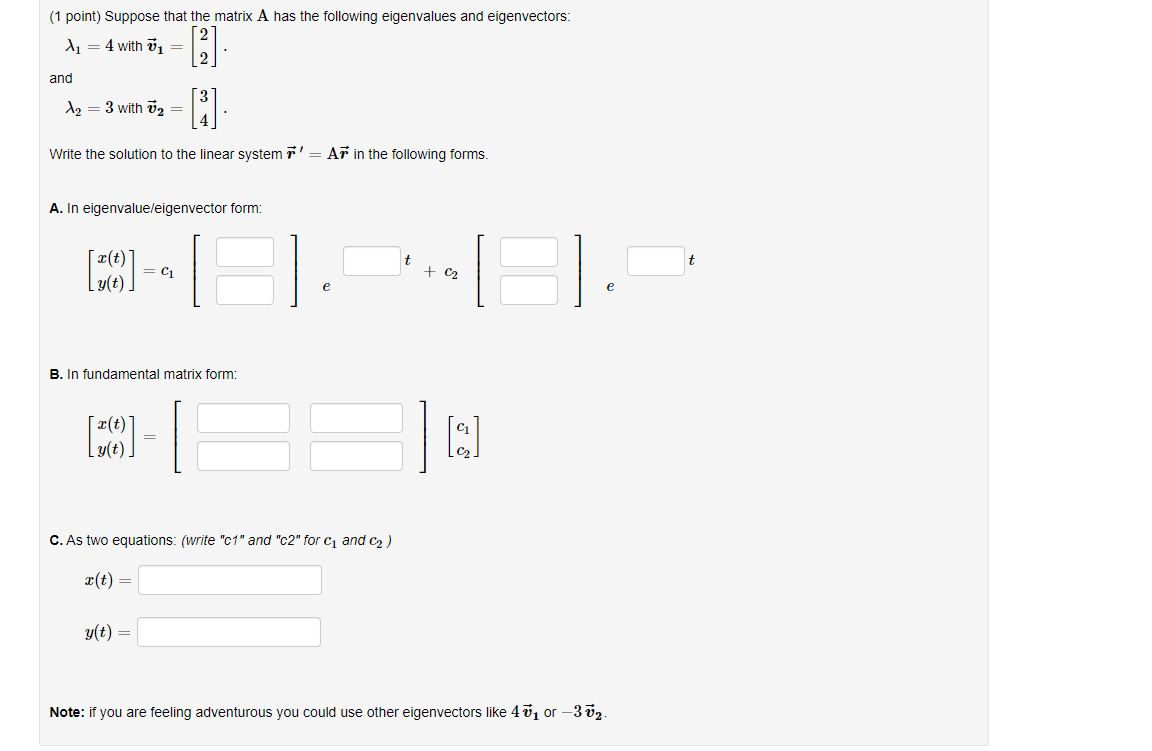(1 point) Suppose that the matrix A has the following eigenvalues and eigenvectors: A1 = 4 with = and  [i] Az = 3 with Ū2 = Write the solution to the linear system r' = Ar in the following forms. A. In eigenvalue/eigenvector form: t (10) -- + C2 e e B. In fundamental matrix form: (39) - g(t). C. As two equations: (write "c1" and "c2" for C and C2) X(t) = g(t) = Note: if you are feeling adventurous you could use other eigenvectors like 40 or-372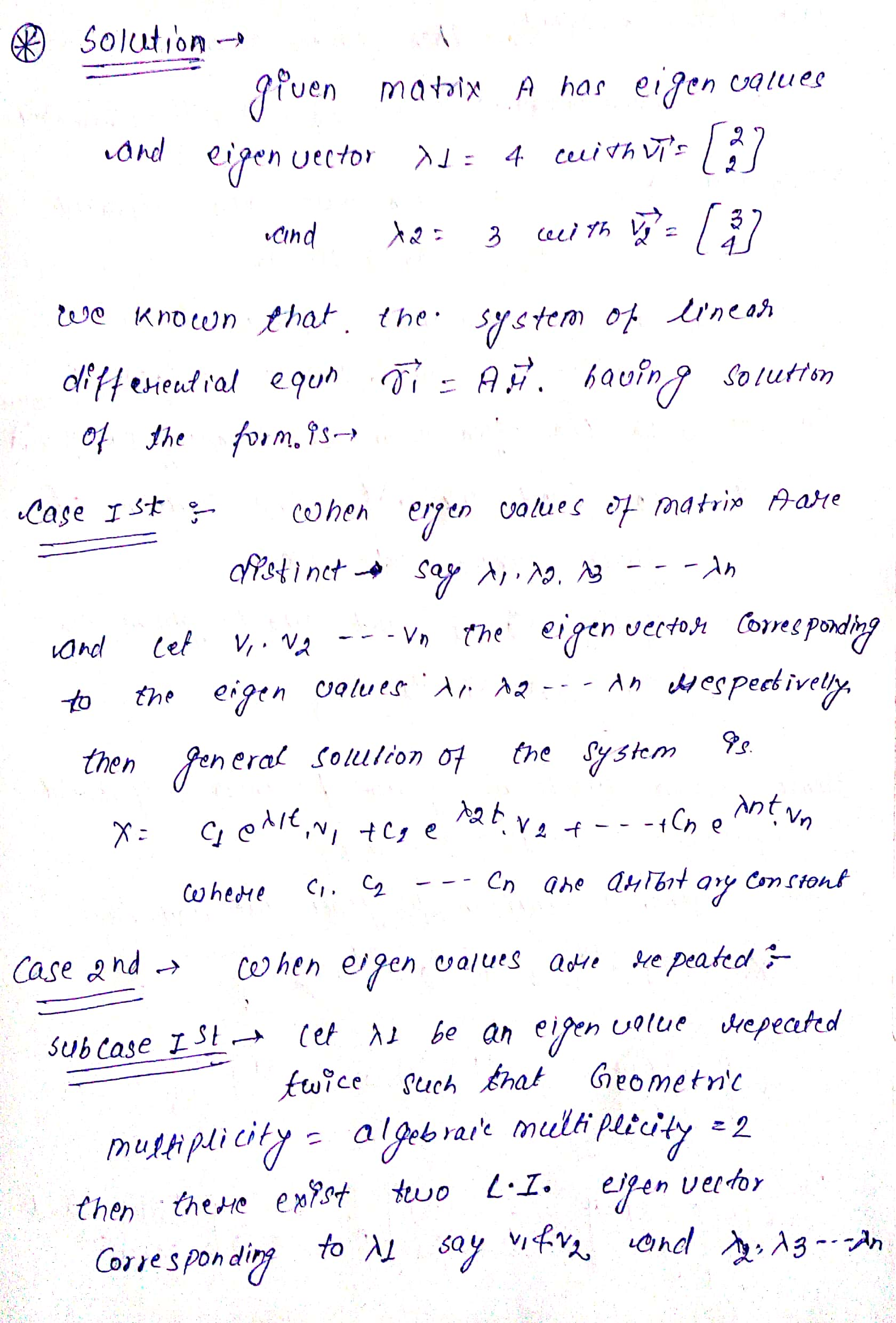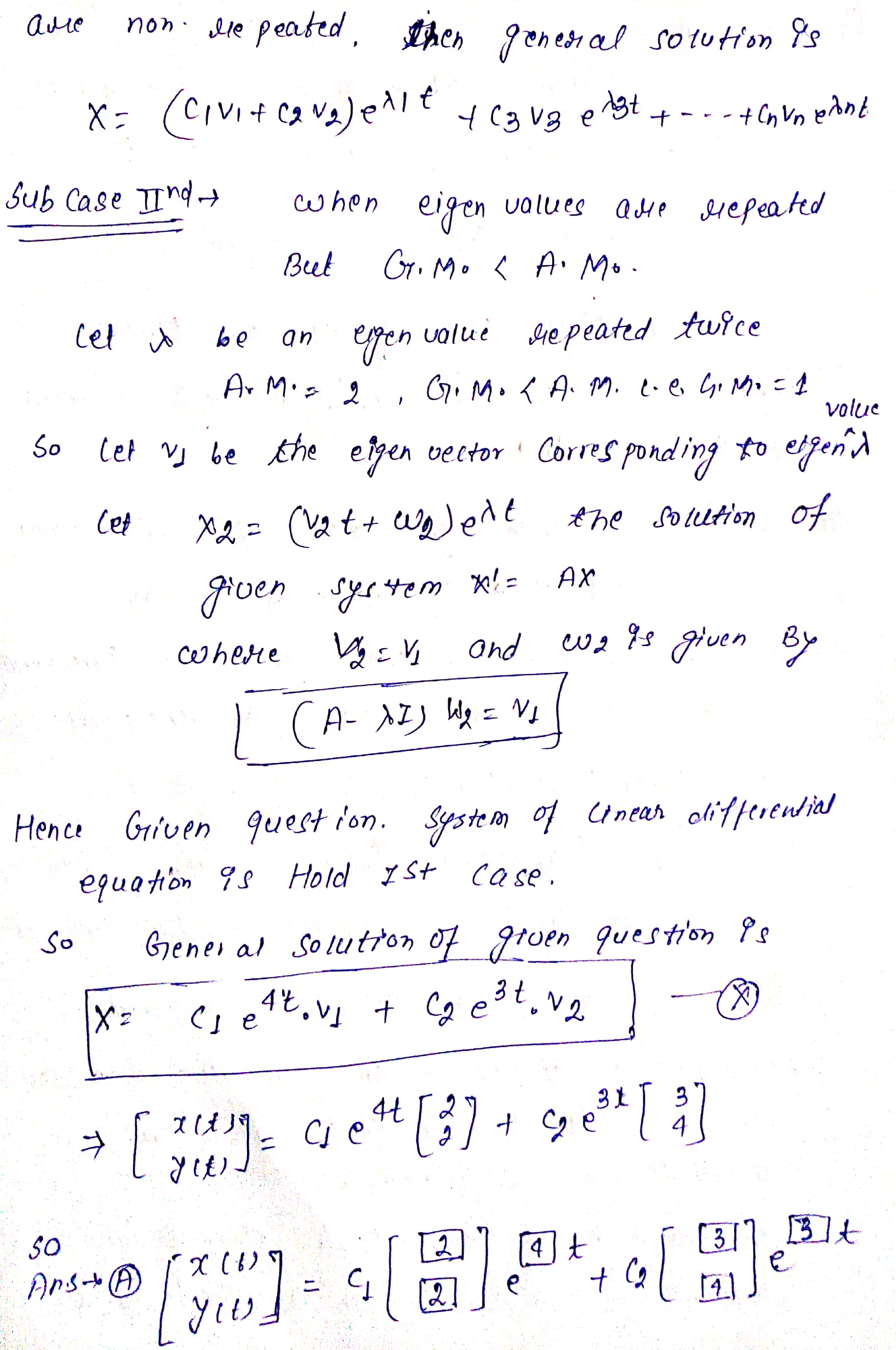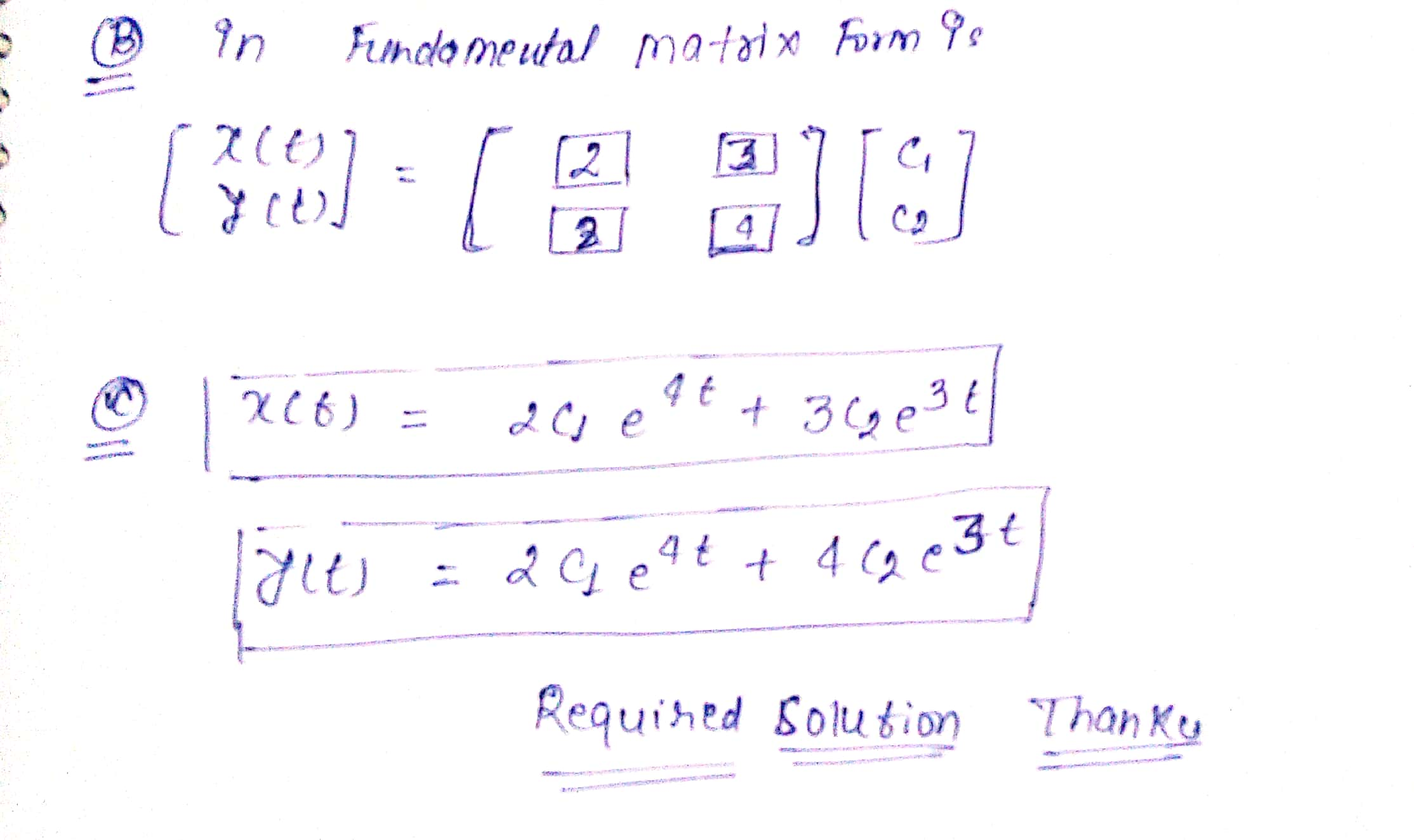##### Add Answer of: (1 point) Suppose that the matrix A has the following eigenvalues and eigenvectors: A1 = 4...
Similar Homework Help Questions
• ### (1 point) Suppose that the matrix A has the following eigenvalues and eigenvectors: 4 = 2...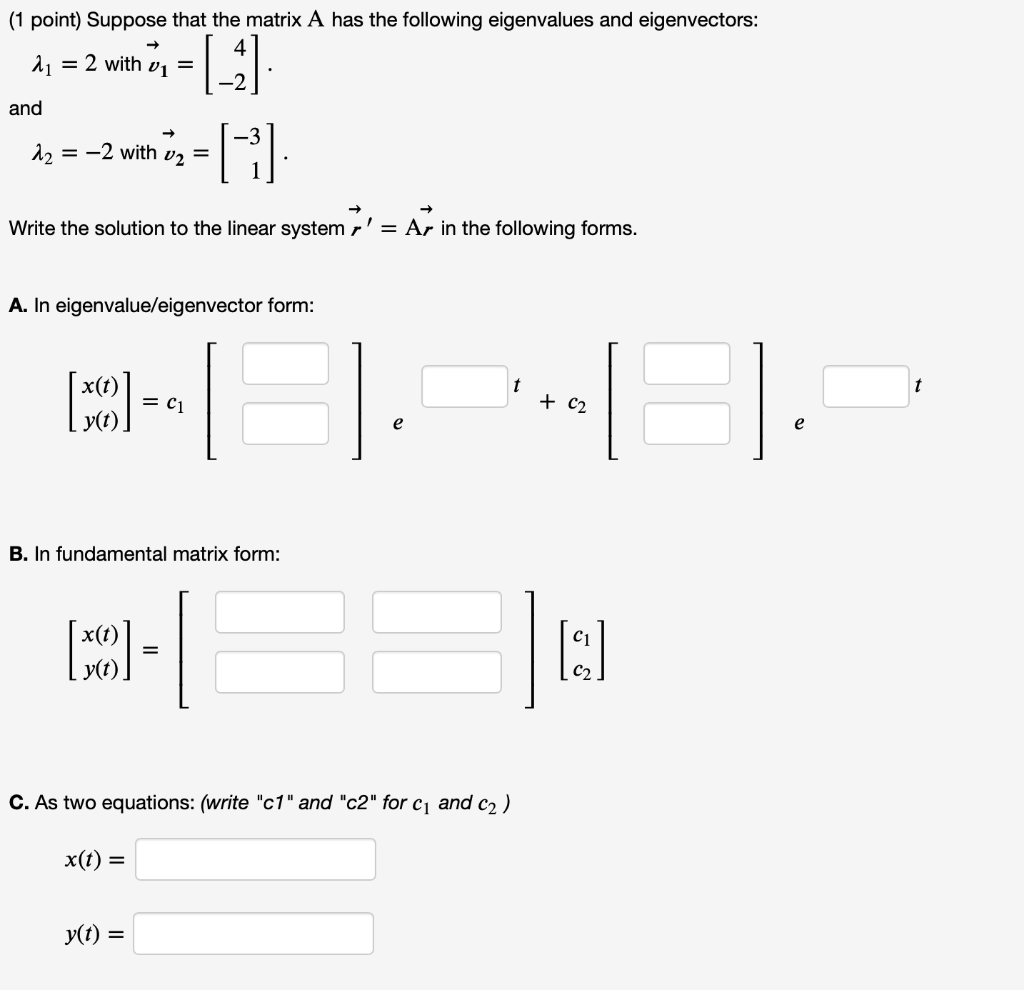(1 point) Suppose that the matrix A has the following eigenvalues and eigenvectors: 4 = 2 with vi = and |_ G 12 = -2 with v2 = Write the solution to the linear system r' = Ar in the following forms. A. In eigenvalue/eigenvector form: x(t) (50) = C1 + C2 e e B. In fundamental matrix form: (MCO) = I: C. As two equations: (write "c1" and "c2" for C1 and c2) x(t) = yt) =

• ### (1 point) Suppose that the matrix A has repeated eigenvalue with the following eigenvector and generalized...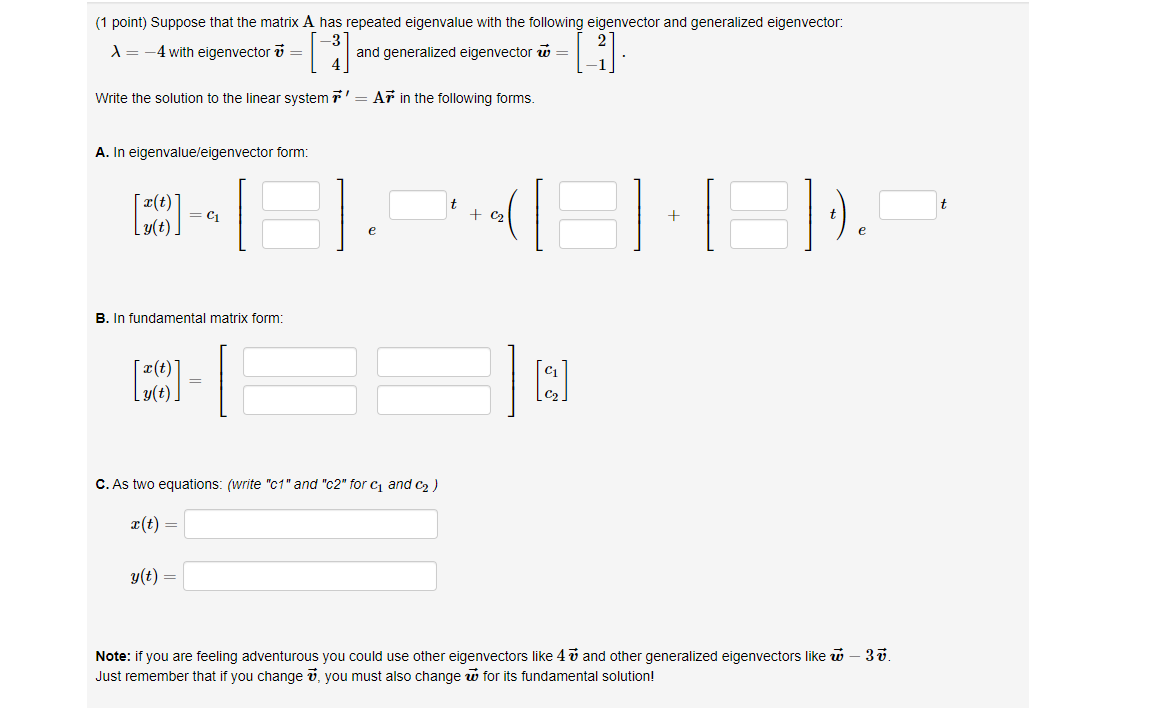(1 point) Suppose that the matrix A has repeated eigenvalue with the following eigenvector and generalized eigenvector: X= -4 with eigenvector v = and generalized eigenvector ū= [] (-1) Write the solution to the linear system r' = Ar in the following forms. A. In eigenvalue/eigenvector form: t t [CO] = C1 + C2 + I g(t). e . - 1 B. In fundamental matrix form: [CO] C. As two equations: (write "c1" and "c2" for 1 and 2) X(t)...

• ### Suppose that the matrix A A has the following eigenvalues and eigenvectors: (1 point) Suppose that...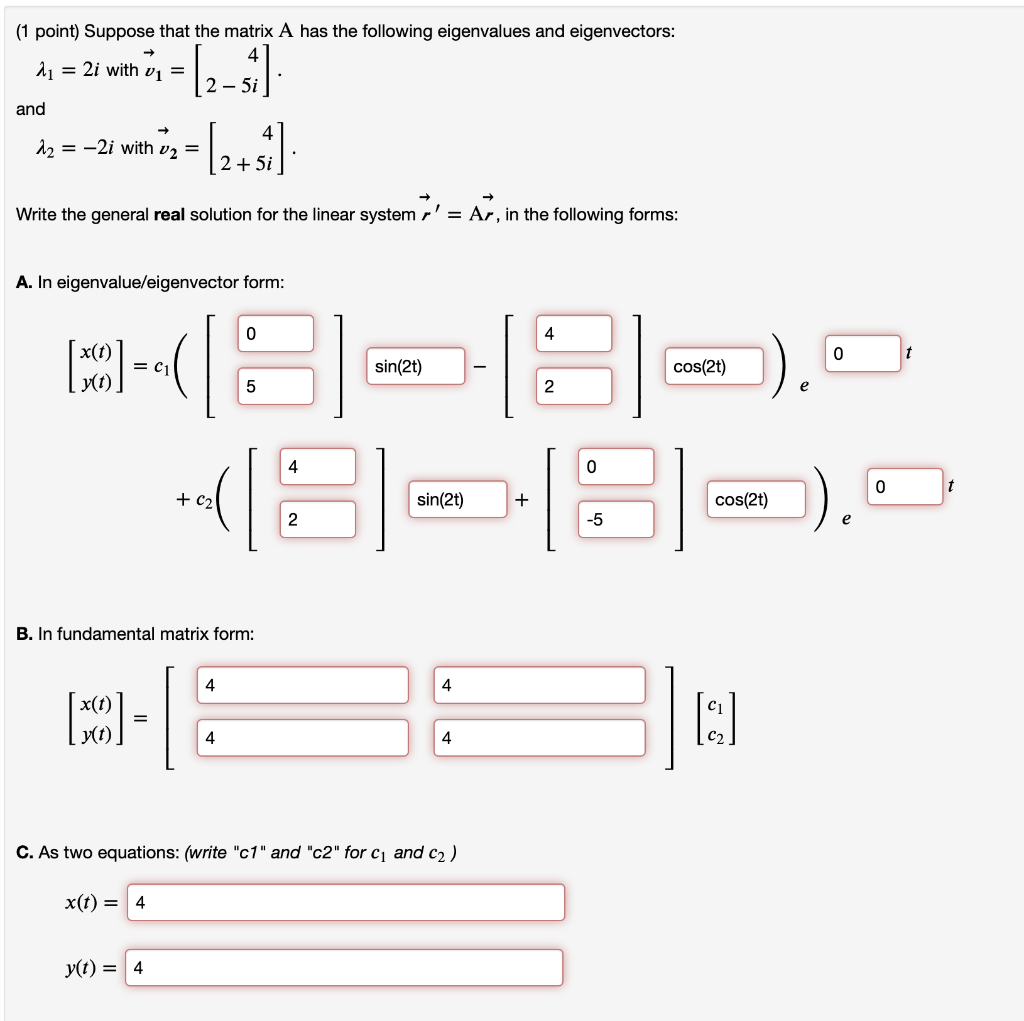Suppose that the matrix A A has the following eigenvalues and eigenvectors: (1 point) Suppose that the matrix A has the following eigenvalues and eigenvectors: 2 = 2i with v1 = 2 - 5i and - 12 = -2i with v2 = (2+1) 2 + 5i Write the general real solution for the linear system r' = Ar, in the following forms: A. In eigenvalue/eigenvector form: 0 4 0 t MODE = C1 sin(2t) cos(2) 5 2 4 0 0...

• ### (1 point) Suppose that the matrix A has the following eigenvalues and eigenvectors 2-2i and -2+2i Write the solution to...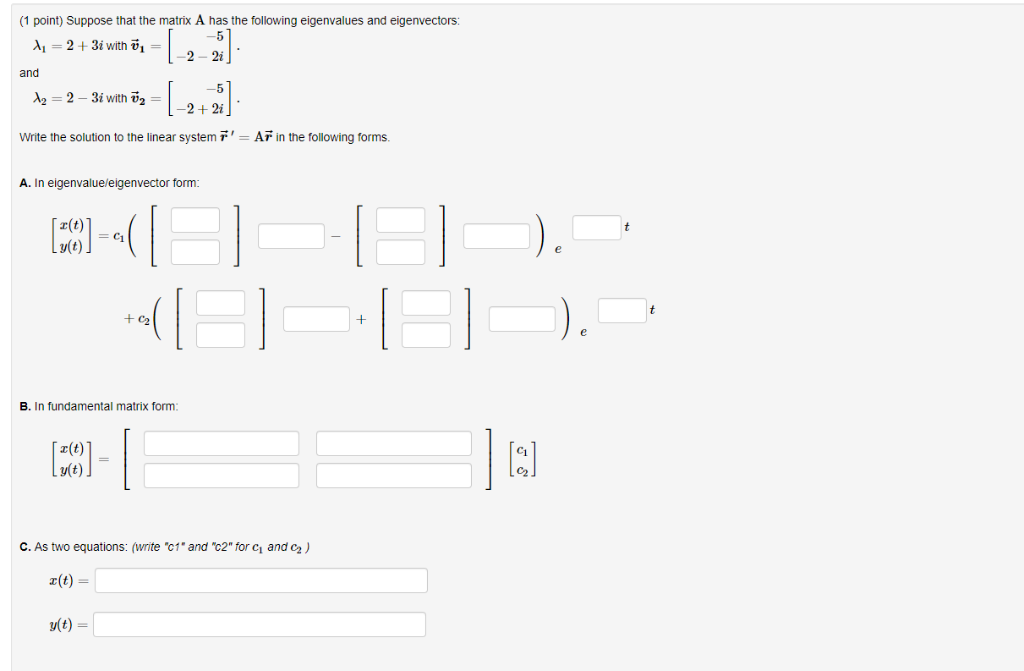(1 point) Suppose that the matrix A has the following eigenvalues and eigenvectors 2-2i and -2+2i Write the solution to the linear system AF in the following forms A. In eigenvalueleigenvector form r(t) B. In fundamental matrix form z(t) v(t) C. As two equations: (write "c1* and "c2" for ci and C2) a(t)- v(t)- (1 point) Suppose that the matrix A has the following eigenvalues and eigenvectors 2-2i and -2+2i Write the solution to the linear system AF in the...

• ### (1 point) Suppose that the matrix A has repeated eigenvalue with the following eigenvector and generalized...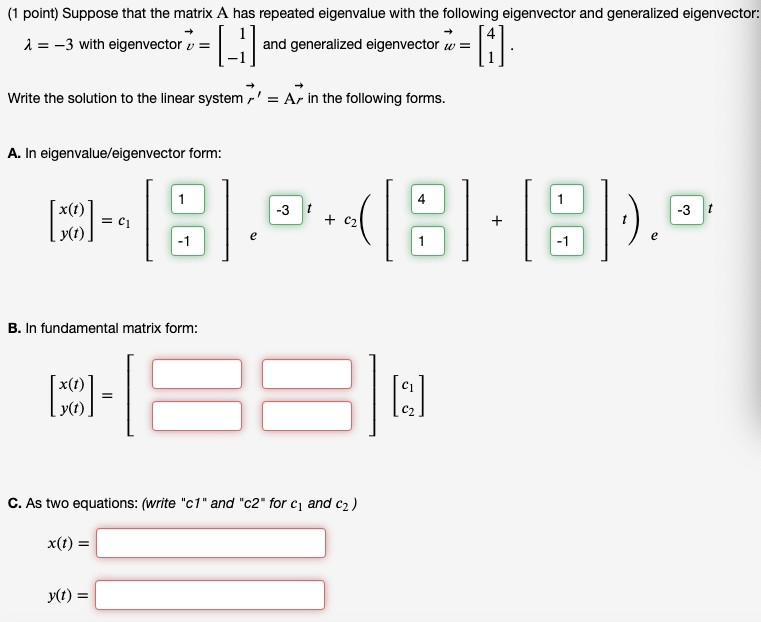(1 point) Suppose that the matrix A has repeated eigenvalue with the following eigenvector and generalized eigenvector: i = -3 with eigenvector v = and generalized eigenvector w= = [-] =  Write the solution to the linear system r' = Ar in the following forms. A. In eigenvalue/eigenvector form: 1 (O) = - 18.05.8:8)... y(t) B. In fundamental matrix form: (O)- x(1) y(t) [:] C. As two equations: (write "c1" and "c2" for c and c2) X(t) = yt)...

• ### (1 point) Consider the initial value problem -2 j' = [ y, y(0) +3] 0 -2...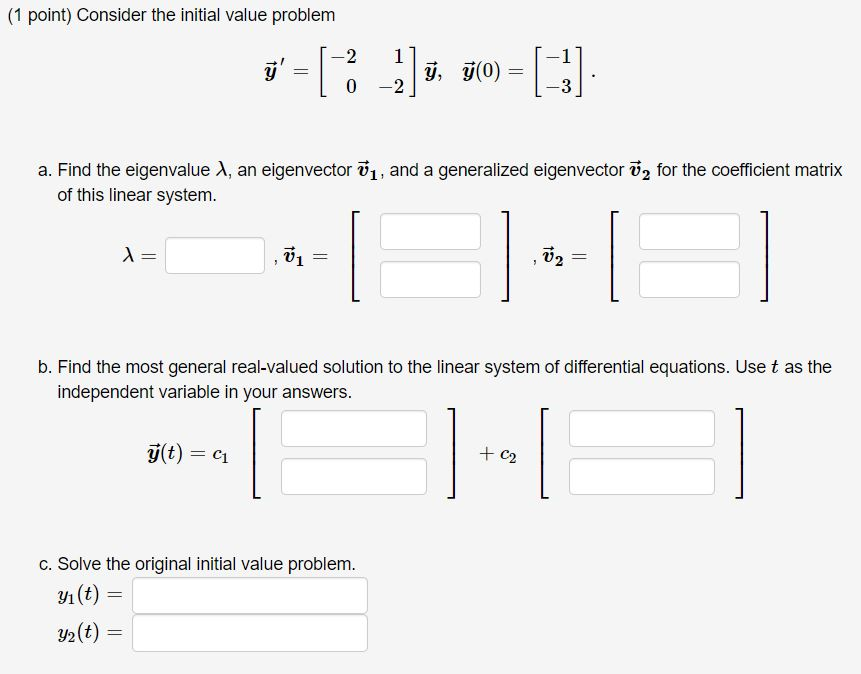(1 point) Consider the initial value problem -2 j' = [ y, y(0) +3] 0 -2 a. Find the eigenvalue 1, an eigenvector 1, and a generalized eigenvector ū2 for the coefficient matrix of this linear system. = --1 V2 = b. Find the most general real-valued solution to the linear system of differential equations. Use t as the independent variable in your answers. g(t) = C1 + C2 c. Solve the original initial value problem. yı(t) = y2(t) ==

• ### a) suppose that the nxn matrix A has its n eigenvalues arranged in decreasing order of absolute size, so that  >>....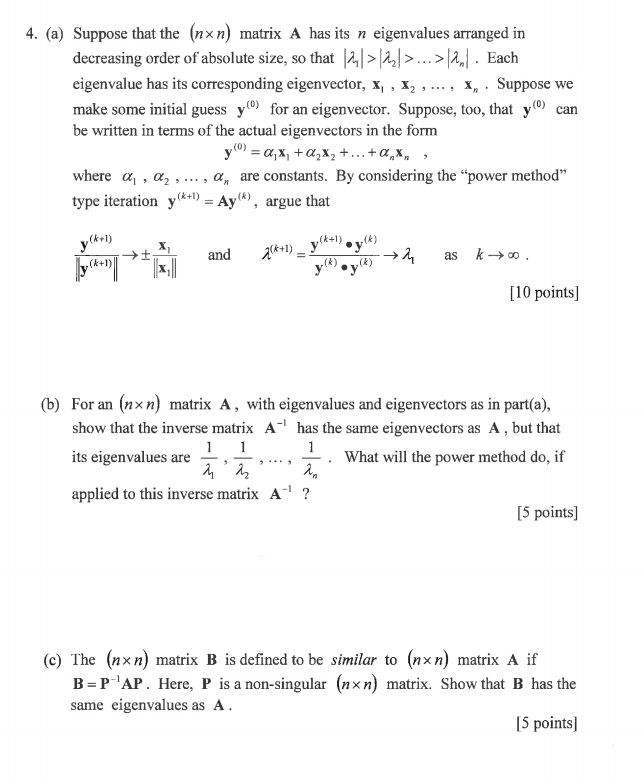a) suppose that the nxn matrix A has its n eigenvalues arranged in decreasing order of absolute size, so that  >>.... each eigenvalue has its corresponding eigenvector, x1,x2,...,xn. suppose we make some initial guess y(0) for an eigenvector. suppose, too, that y(0) can be written in terms of the actual eigenvectors in the form y(0)=alpha1.x1 +alpha2.x2 +...+alpha(n).x(n), where alpha1, alpha2, alpha(n) are constants. by considering the "power method" type iteration y(k+1)=Ay(k) argue that (see attached image) b) from an nxn...

• ### 5. The following matrix B has known eigenvalues λ1-1 and λ2-6. 10a-1 B-0b-23 c30 0 Where...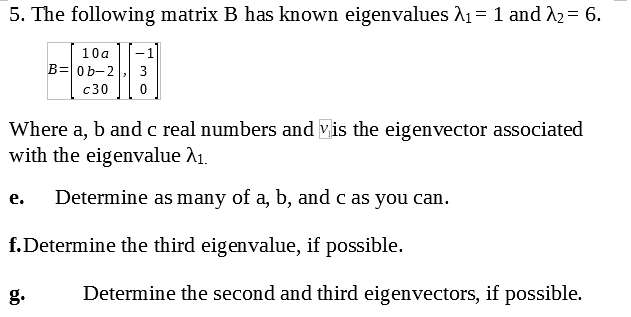5. The following matrix B has known eigenvalues λ1-1 and λ2-6. 10a-1 B-0b-23 c30 0 Where a, b and c real numbers and vis the eigenvector associated with the eigenvalue A1. e. Determine as many of a, b, and c as you can. f.Determine the third eigenvalue, if possible. g.Determine the second and third eigenvectors, if possible.

• ### (1 point) Given that ū = and are eigenvectors of the matrix -12 24 determine the...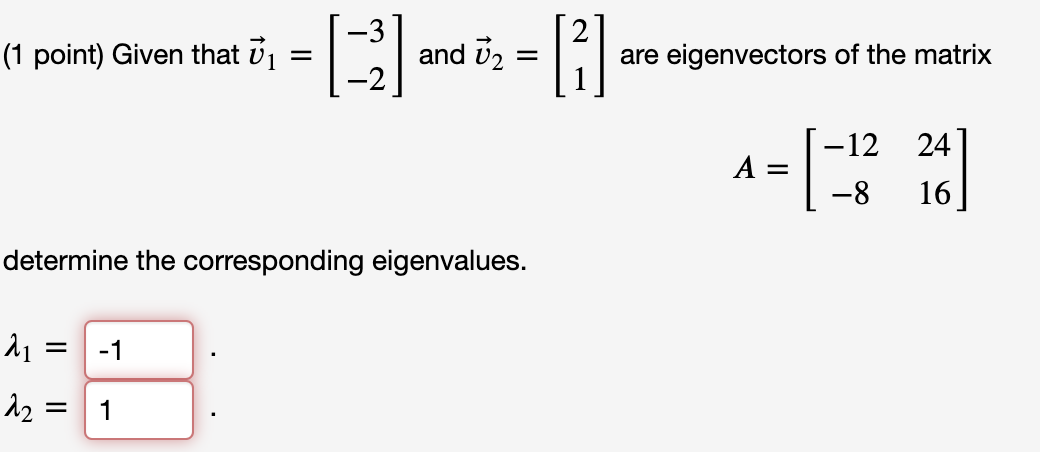(1 point) Given that ū = and are eigenvectors of the matrix -12 24 determine the corresponding eigenvalues. 21 = -1 12 = 1 (1 point) Solve the system -6 1 dx dt х -6 -1 with the initial value 0 x(0) = -2 x(t) = (1 point) Calculate the eigenvalues of this matrix: [Note-- you'll probably want to use a calculator or computer to estimate the roots of the polynomial which defines the eigenvalues. You also may want to...

• ### linear algebra Show that any two eigenvectors of the symmetric matrix corresponding to distinct eigenvalues are...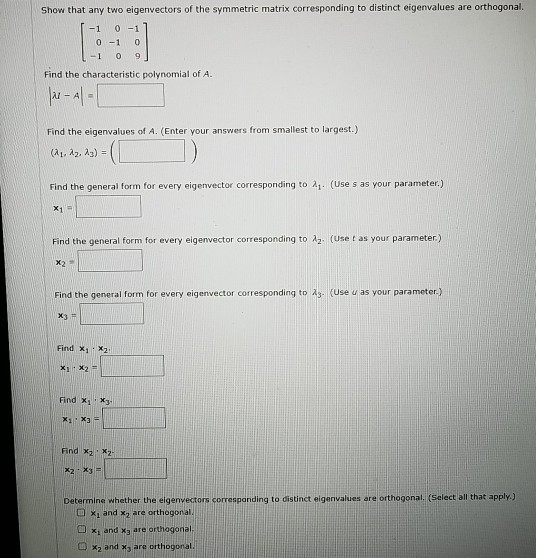linear algebra Show that any two eigenvectors of the symmetric matrix corresponding to distinct eigenvalues are orthogonal -1 0-1 0-1 0 - 1 0 9 1 Find the characteristic polynomial of A. |x - Al- Find the eigenvalues of A. (Enter your answers from smallest to largest.) (21, 22, 23) Find the general form for every eigenvector corresponding to 21. (Uses as your parameter.) X1 - Find the general form for every elge vector corresponding to Az. (Uset as your...

Free Homework App